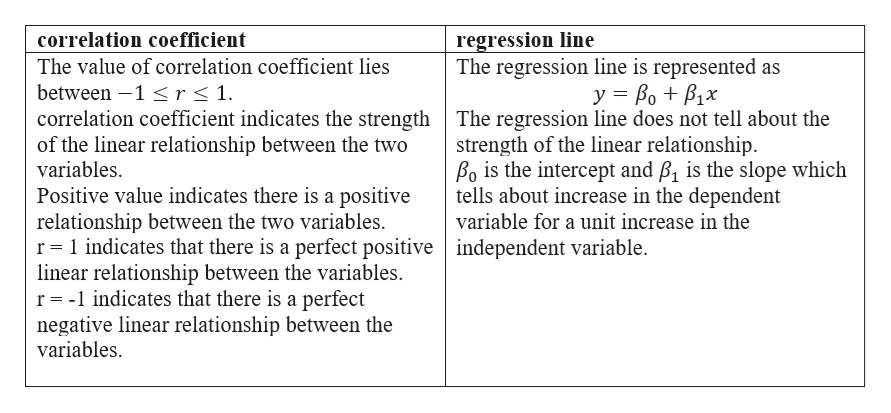# What is the difference between the correlation coeffecient and the regression line in a scatterplot?

Question
16 views

What is the difference between the correlation coeffecient and the regression line in a scatterplot?

check_circle

Step 1

Difference between correlation coefficient and reg...help_outlineImage Transcriptioncloseregression line The regression line is represented as correlation coefficient The value of correlation coefficient lies between 1 r < 1. correlation coefficient indicates the strength The regression line does not tell about the of the linear relationship between the two variables. y Bo B1x strength of the linear relationship Bo is the intercept and B1 is the slope which tells about increase in the dependent variable for a unit increase in the Positive value indicates there is a positive relationship between the two variables r 1 indicates that there is a perfect positive independent variable. linear relationship between the variables. r -l indicates that there is a perfect negative linear relationship between the variables fullscreen

### Want to see the full answer?

See Solution

#### Want to see this answer and more?

Solutions are written by subject experts who are available 24/7. Questions are typically answered within 1 hour.*

See Solution
*Response times may vary by subject and question.
Tagged in

### Other